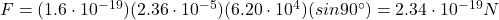## slader A long, straight wire carries a current of 5.20 AA. An electron is traveling in the vicinity of the wire. At the instant when the ele

Question

slader A long, straight wire carries a current of 5.20 AA. An electron is traveling in the vicinity of the wire. At the instant when the electron is 4.40 cmcm from the wire and traveling with a speed of 6.20×104 m/sm/s directly toward the wire, what is the magnitude of the force that the magnetic field of the current exerts on the electron?

in progress 0
2 weeks 2021-08-29T19:02:02+00:00 1 Answers 0 views 0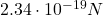Explanation:

The strength of the magnetic field produced by a current-carrying wire is: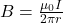where: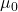is the vacuum permeability

I is the current in the wire

r is the distance from the wire

In this case,

I = 5.20 A

r = 4.40 cm = 0.044 m

Therefore,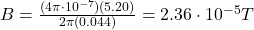The direction of the field is along concentric circles centered in the wire.

The force exerted by a magnetic field on a moving charged particle is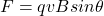where

q is the charge

v is the velocity of the particle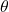is the direction between v and B

In this problem: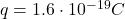is the magnitude of the charge of the particle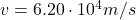is the velocity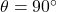, because the electron is travelling towards the wire, so perpendicular to the lines of the magnetic field

Therefore, the magnitude of the force is: